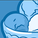Announcements
Applications are open for the 2024 Qlik Luminary Program. Apply by December 15 here.
cancel
Showing results for
Did you mean:Contributor II

## KPI Counting Aggregated Deltas

Hey All,

This is my first time posting -- please let me know if I'm doing anything wrong.

I have a tricky bit of aggregation that I just can’t figure out. I have a dataset with values and dates that I append to each month in a qvd. The data looks like this:

 ID Amount Report_Date A 100 1/1/2020 B 200 1/1/2020 C 300 1/1/2020 D 400 1/1/2020 A 200 2/1/2020 B 200 2/1/2020 D 200 2/1/2020 E 500 2/1/2020

I am aggregating sums based on MIN and MAX dates for my chart. I get the Amount Changed by subtracting the Beginning Balance from the Ending Balance. Like this:

 ID Beginning Balance Amount Changed Ending Balance A 100 100 200 B 200 0 200 C 300 -300 0 D 400 -200 200 E 0 500 500

The formulas I’m using look like this:

Beginning Balance: Sum({<Report_Date = {'\$(=date(Min(Report_Date)))'}>}[Amount])

Ending Balance: Sum({<Report_Date = {'\$(=date(Max(Report_Date)))'}>}[Amount])

Amount Changed: Sum({<Report_Date = {'\$(=date(Max(Report_Date)))'}>}[Amount]) - Sum({<Report_Date = {'\$(=date(Min(Report_Date)))'}>}[Amount])

These formulas are working fine. Each positive change (>0) represents a new expense, and each negative change (<0) represents a refunded expense. I want KPIs counting how many IDs have a positive change amount and a negative change amount, but I can’t figure out how to write the formula that says ‘Count all of the IDs where Amount Changed is >0.’ I end up with a formula with nested aggregations and sets and Qlik doesn’t like it.

Any idea of how I can write a KPI that counts the positive and negative deltas between the Beginning and Ending Balances?

1 Solution

Accepted SolutionsSpecialist

Try this:

count( distinct if(aggr(Sum({<Report_Date = {'\$(=date(Max(Report_Date)))'}>}[Amount]) - Sum({<Report_Date = {'\$(=date(Min(Report_Date)))'}>}[Amount]), ID)>0,ID))

2 RepliesSpecialist

Try this:

count( distinct if(aggr(Sum({<Report_Date = {'\$(=date(Max(Report_Date)))'}>}[Amount]) - Sum({<Report_Date = {'\$(=date(Min(Report_Date)))'}>}[Amount]), ID)>0,ID))Contributor II
Author

That did the trick -- pure genius! Thank you!Tags
Community Browser